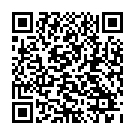## Y6 - Fractions (including Decimals and Percentages) - Interactive Quiz

### Related Worksheets

An interactive quiz which test your understanding of all the Number – Addition, Subtraction, Multiplication and Division objectives in the Year 6 curriculum.

Alternatively, you can use the Interactive Maths Quiz which includes objectives from all strands of the Year 6 curriculum.

This quiz tests the following objectives:

• use common factors to simplify fractions; use common multiples to express fractions in the same denomination
• compare and order fractions, including fractions >1
• add and subtract fractions with different denominators and mixed numbers, using the concept of equivalent fractions
• multiply simple pairs of proper fractions, writing the answer in its simplest form [for example,×]
• divide proper fractions by whole numbers [for example,÷ 2 =]
• associate a fraction with division and calculate decimal fraction equivalents [for example, 0.375] for a simple fraction [for example,]
• identify the value of each digit in numbers given to 3 decimal places and multiply and divide numbers by 10, 100 and 1,000 giving answers up to 3 decimal places
• multiply one-digit numbers with up to 2 decimal places by whole numbers
• use written division methods in cases where the answer has up to 2 decimal places
• solve problems which require answers to be rounded to specified degrees of accuracy
• recall and use equivalences between simple fractions, decimals and percentages, including in different contextsScan to open this game on a mobile device. Right-click to copy and paste it onto a homework sheet.